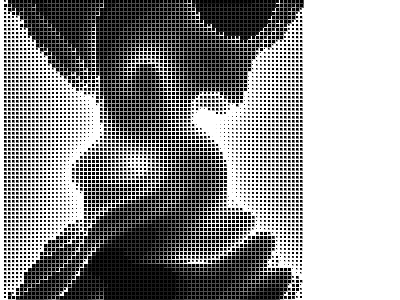# TEA STORM

814 words ~ 4-8 minsMathieu 'p01' Henri on September 14th, 2013

# TEA STORM

256 bytes JavaScript signed distance field raymarcher using 2D Canvas. TEA STORM won at Function 2013You've seen distance field raymarching here before with JSpongy, Hypersonic Mandelbulb and ANDES to name a few. This technique championned by Iñigo Quilez of Elevated and Brave fame is perfect to render complex shapes in a few kilo bytes or even hundred bytes, but only a handful of size optimizers extraordinaire did raymarching in 256 or even 128 bytes.

## Source code

``<body onload=setInterval("for(t-=.1,x=h,c.height=300,Q=Math.cos;x--;)for(y=h;y--;c.getContext('2d').fillRect(x*4,y*4,N,N))for(N=D=4;X=D*x/h-D/2,Y=D*y/h-D/2,Z=D/2-9,D+=d=(X*X+Y*Y*Q(t/6+Q(D-X-Y))+Z*Z)/9-1+Q(X+t)*Q(Y-t),.1<d*N;)N-=.1",t=h=75)><canvas id=c>``

And voilà, 253 bytes of raymarching goodness.

## How does it work ?

This time, this is the real deal: No fixed step raymarching but a distance function that gives an estimate of how far is the surface of the object at each point in 3D space and the rays march on until they get close enough.

### The setup and loop

That part is trivial: A `canvas`, a `body` element with `onload` event setting a timer that clears the canvas, adjust the time variable, go through each pixel and render them. Just make sure to reuse variables where possible, set the right properties and create alias variables where necessary.

``<body onload=setInterval("for(t-=.1,x=h,c.height=300,Q=Math.cos;x--;)for(y=h;y--;/* draw */)/* compute */",t=h=75)><canvas id=c>``

Once more with tons of comments:

``<body onload=setInterval("/* X loop from 75 to 0 */for(/* adjust the time variable */t-=.1,x=h,/* clear the canvas and size it to 300x300 i.e. 75*4, remember that the default resolution of a Canvas is 300x150 */c.height=300,/* alias for Math.cos */Q=Math.cos;x--;)/* Y loop from 75 to 0 */for(y=h;y--;/* draw the pixel(x,y) */)/* compute the intensity of the pixel(x, y) */",t=h=75)><canvas id=c>``

MINI DISTRICT is actually a 2.5D effect; casts 150 rays checking at most 150 position along the rays which amount to 150x150 = 22.500 tests.

TEA STORM on the other hand is purely a 3D effect; casts 75x75 rays with up to 40 checks along the rays which amounts to a maximum of 75x75x40 = 225,000 tests.

That is a 10x more calculations. But the good news is that the distance function helps a lot here and things are not as dire as they seemed.

The 75x75 rays are blown up 4 times to fill up a 300x300 pixels canvas.

The default `fillStyle` of Canvas is black and there is simply no bytes to waste on changing that. Instead the shades of grey are done by drawing axis aligned square with fractional size. This introduces anti-aliasing which results in various shades of grey instead of plain black and white. Simple.

``````c.getContext('2d').fillRect(x*4,y*4,N,N)
// with N in the range [0; 4]``````

### The camera

This time around, the camera is static. The object evolves over time because we tweak the distance function. The origin of the camera lies in {0, 0, -9} and looks toward {0, 0, 0} like this:

``````for(x=h;x--;)
for(y=h;y--;/* draw the shade of grey */)
for(N=D=4;
X=D*x/h-D/2,
Y=D*y/h-D/2,
Z=D/2-9,
/* here be dragons and distance function */``````

The variable `D` represents how far we marched along the current camera ray.

### The distance function

What you see in TEA STORM is basically a sphere. A crazy twisted sphere, and here is the breakdown of the distance function and how this little monster came to life:

``````// Sphere
d=(X*X+Y*Y+Z*Z)/9-1
// Sphere morphing into a cylinder
d=(X*X+Y*Y*Q(t/6)+Z*Z)/9-1
// Bumpy sphere
d=(X*X+Y*Y+Z*Z)/9-1+Q(X+t)*Q(Y-t)
// Bumpy sphere-cylinder
d=(X*X+Y*Y*Q(t/6)+Z*Z)/9-1+Q(X+t)*Q(Y-t)

// Bumpy twirling morphing sphere-cylinder \(';;')/
d=(X*X+Y*Y*Q(t/6+Q(D-X-Y))+Z*Z)/9-1+Q(X+t)*Q(Y-t)``````

The loop for each ray look like this:

``````for(N=D=4;X=D*x/h-D/2,Y=D*y/h-D/2,Z=D/2-9,D+=d=(X*X+Y*Y*Q(t/6+Q(D-X-Y))+Z*Z)/9-1+Q(X+t)*Q(Y-t),.1<d*N;)
N-=.1``````

As you can see, `N`, the number of iterations, and `D` the distance, start at 4. This is a safe distance considering the origin of the camera. At each step, the new position along the camera ray is computed in 3D space in `X`, `Y` and `Z`, the distance to the object is estimated in `d` and added to `D`, and `N` decreased by 0.1.

The loop stops when the condition `.1<d*N` is true. This condition does two things:

• Ensure that we stop when `N` reaches 0 or we have reached the object.
• The multiplication `d*N` introduces an approximation of the focal distance.

This means that the further we are from the origin of the camera, the bigger the margin of of error we can allow without any visual artefact. Of course this is not entirelly correct since `N` does not represent the distance travelled but the number of iterations, but this is good enough.

## Karma

TEA STORM won at the 256 bytes intro competition at Function 2013 in Budapest, Hungary on September 14th, 2013. As usual for my demoscene productions, TEA STORM is available on Pouet.net where any comments and gestures are appreciated.

Hope you like the little storm and write up.